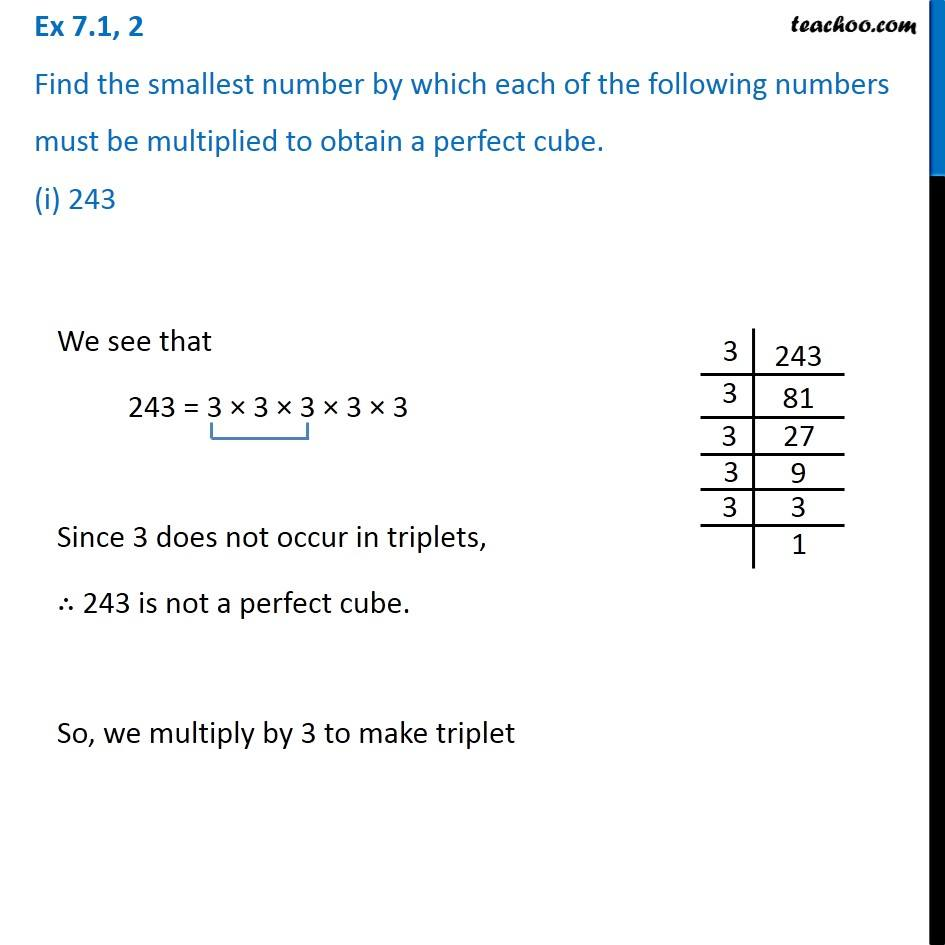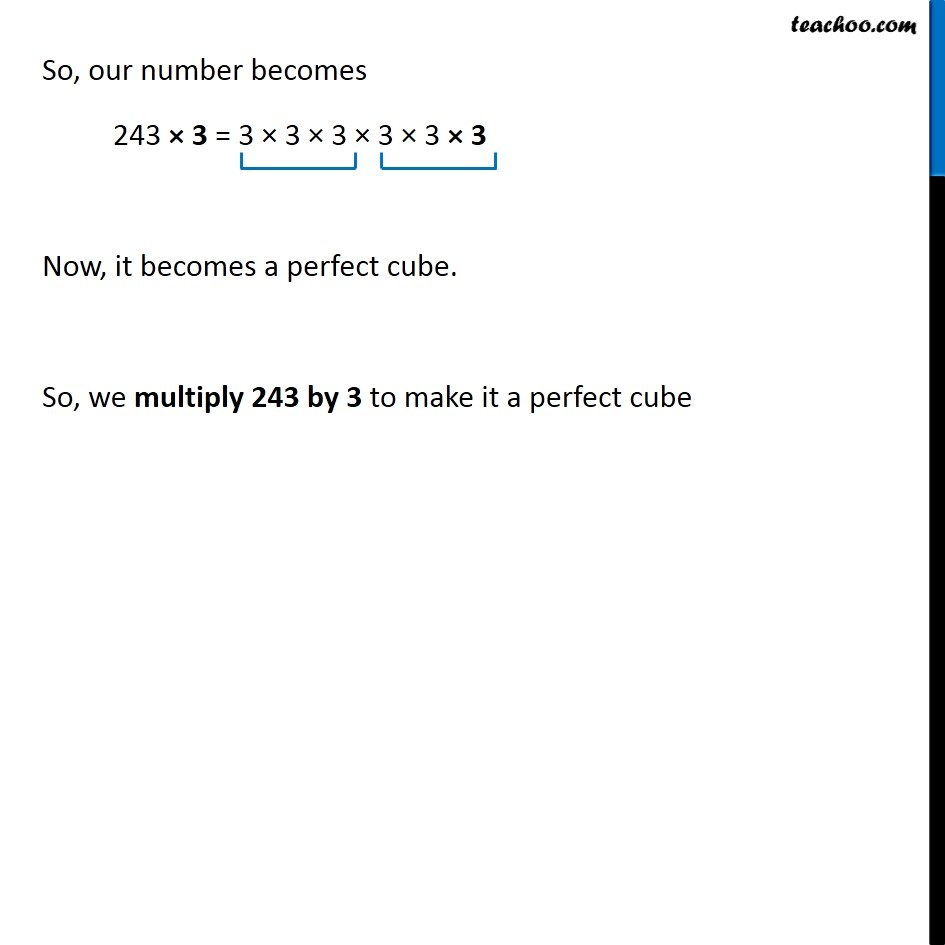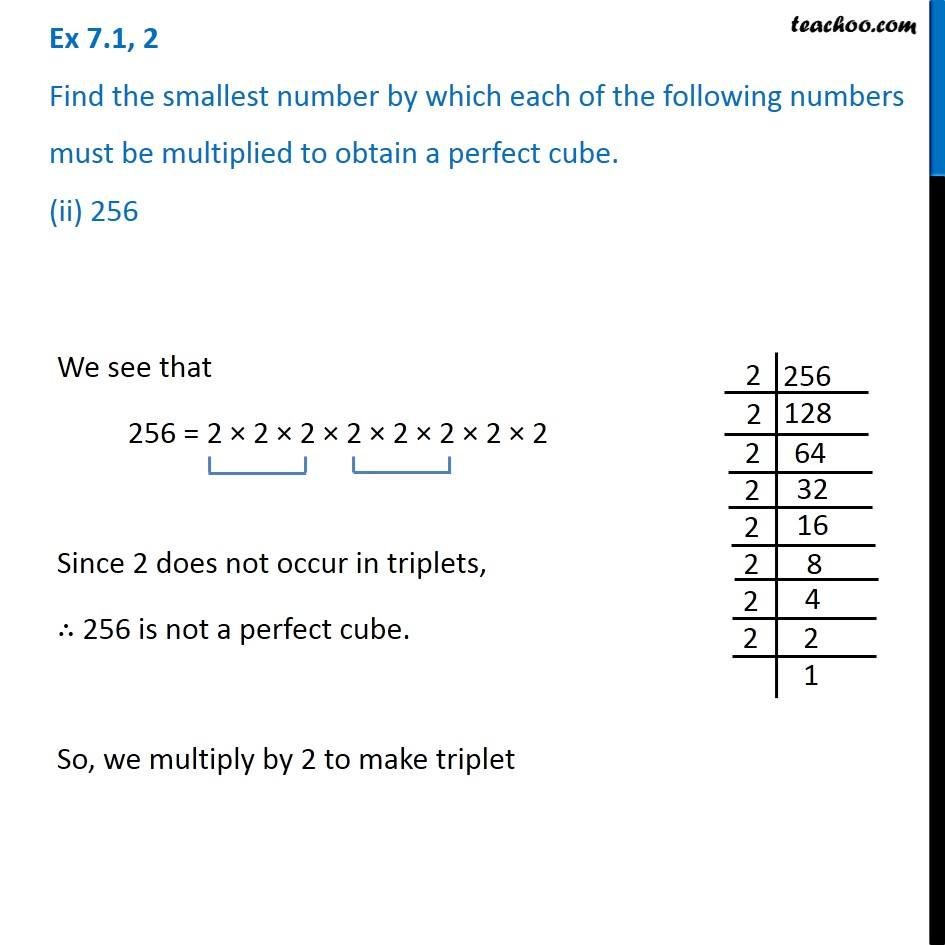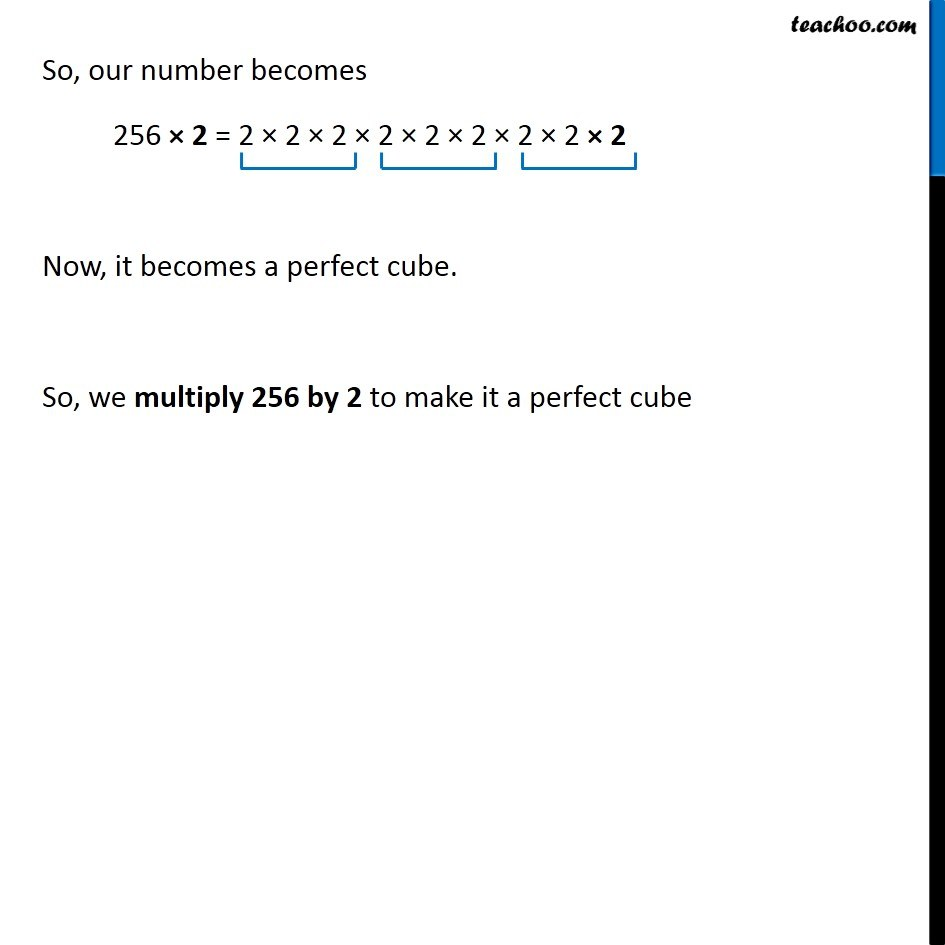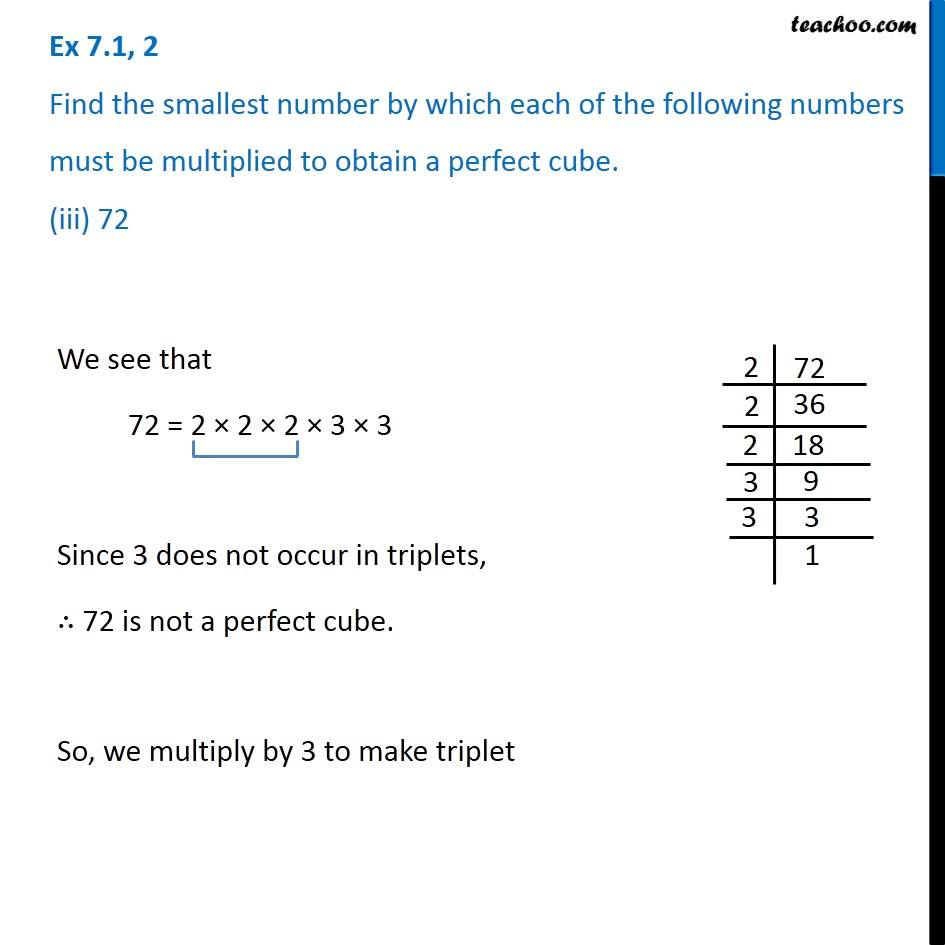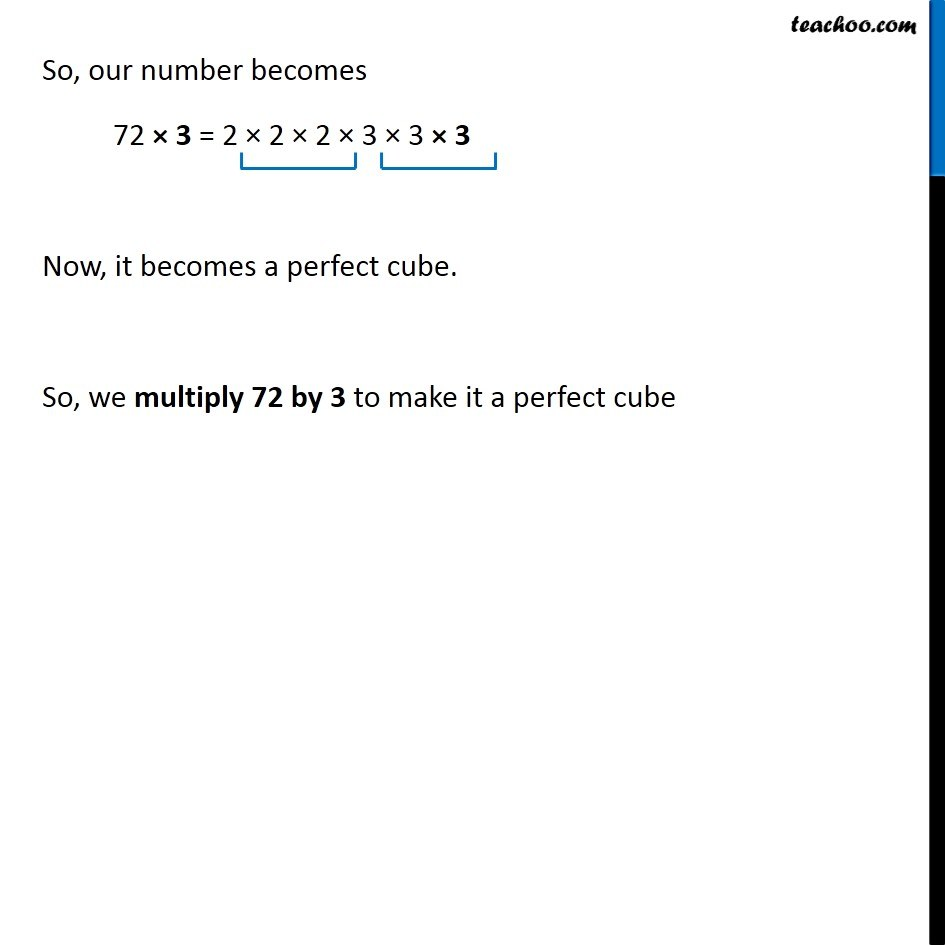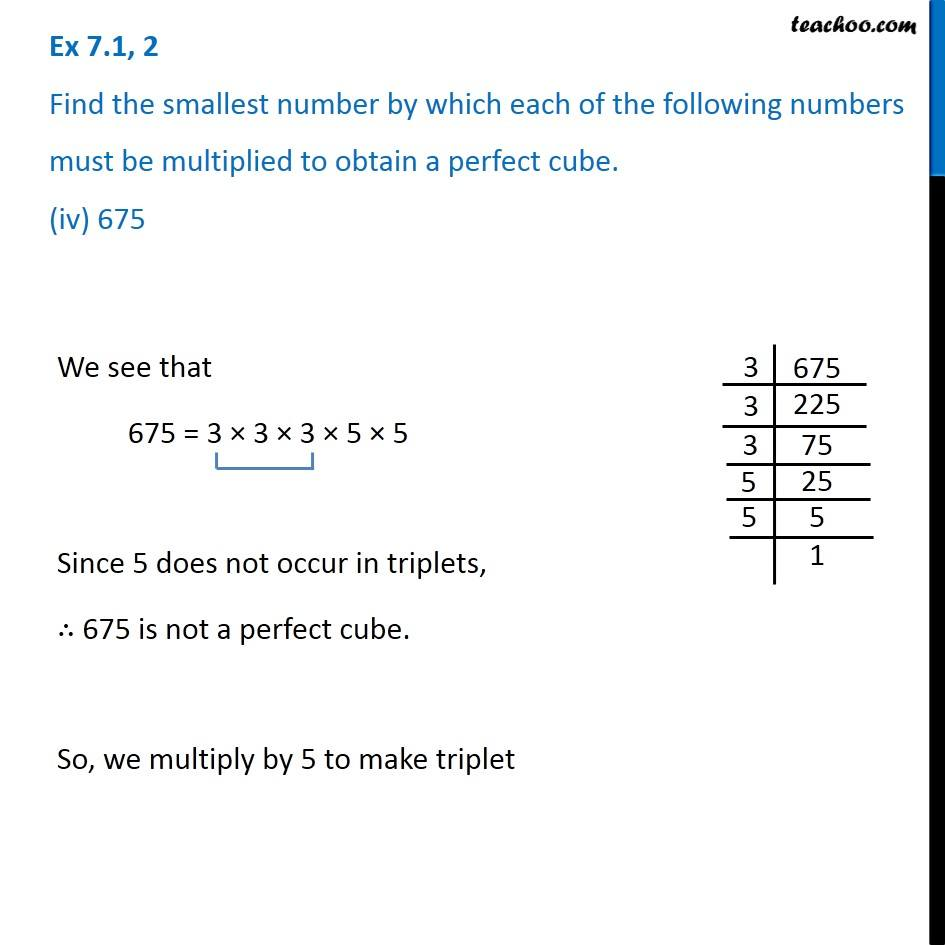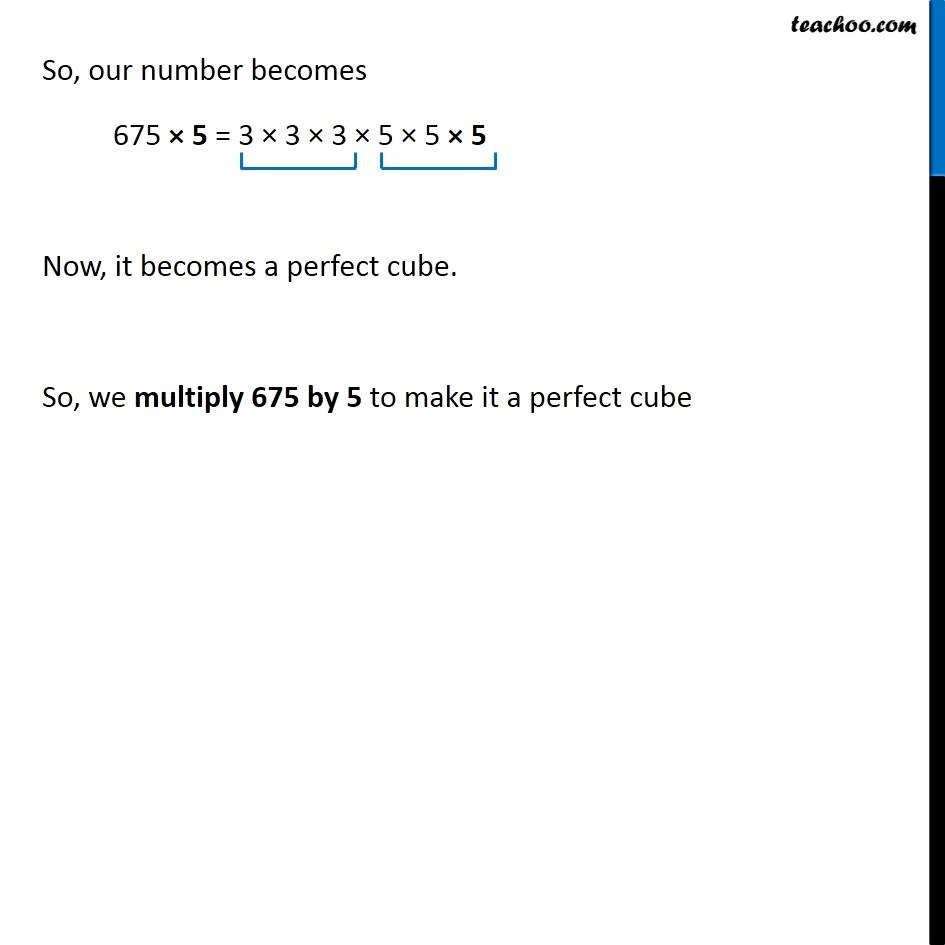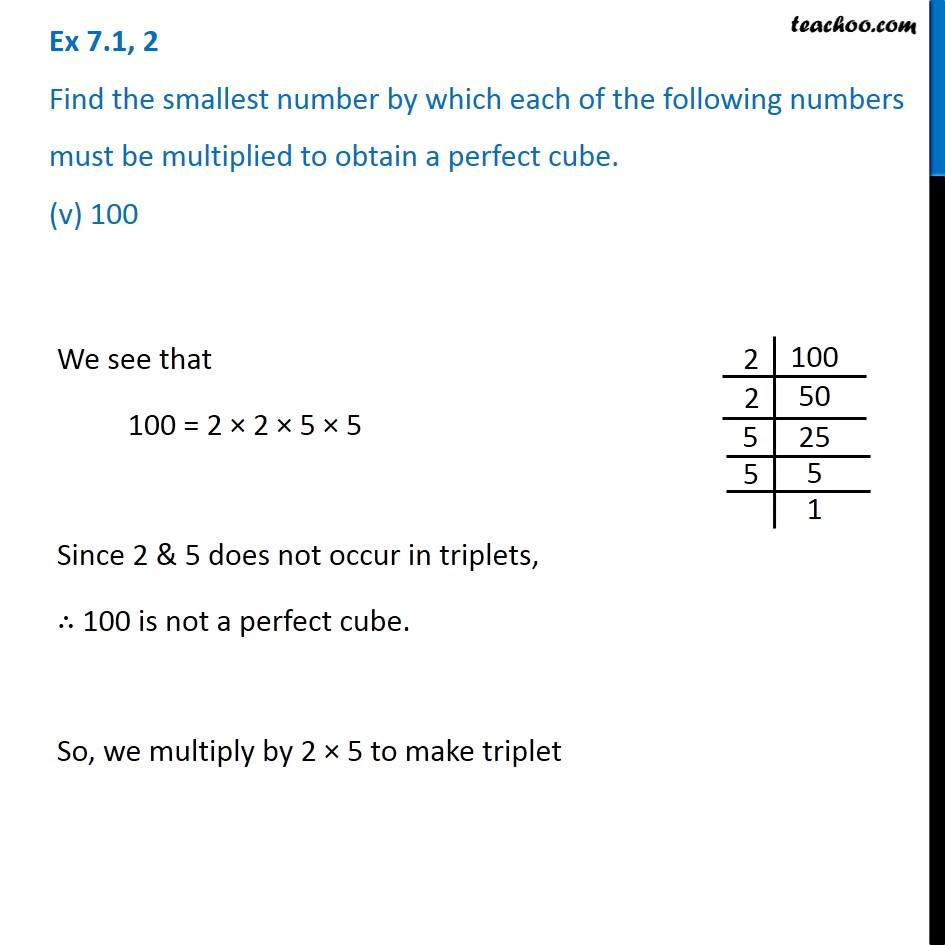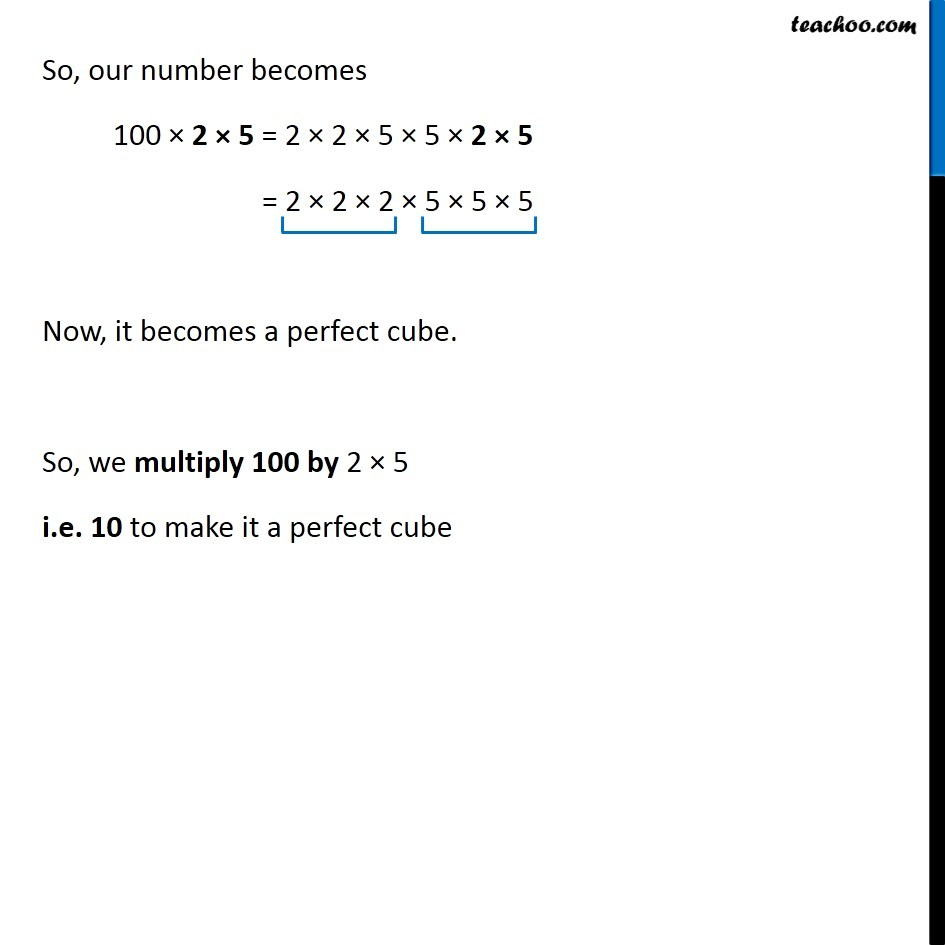Subscribe to our Youtube Channel - https://you.tube/teachoo

1. Chapter 7 Class 8 Cubes and Cube Roots
2. Concept wise
3. Smallest number multiplied to get a perfect cube

Transcript

Ex 7.1, 2 Find the smallest number by which each of the following numbers must be multiplied to obtain a perfect cube. (i) 243 We see that 243 = 3 × 3 × 3 × 3 × 3 Since 3 does not occur in triplets, ∴ 243 is not a perfect cube. So, we multiply by 3 to make triplet So, our number becomes 243 × 3 = 3 × 3 × 3 × 3 × 3 × 3 Now, it becomes a perfect cube. So, we multiply 243 by 3 to make it a perfect cube Ex 7.1, 2 Find the smallest number by which each of the following numbers must be multiplied to obtain a perfect cube. (ii) 256 We see that 256 = 2 × 2 × 2 × 2 × 2 × 2 × 2 × 2 Since 2 does not occur in triplets, ∴ 256 is not a perfect cube. So, we multiply by 2 to make triplet So, our number becomes 256 × 2 = 2 × 2 × 2 × 2 × 2 × 2 × 2 × 2 × 2 Now, it becomes a perfect cube. So, we multiply 256 by 2 to make it a perfect cube Ex 7.1, 2 Find the smallest number by which each of the following numbers must be multiplied to obtain a perfect cube. (iii) 72 We see that 72 = 2 × 2 × 2 × 3 × 3 Since 3 does not occur in triplets, ∴ 72 is not a perfect cube. So, we multiply by 3 to make triplet So, our number becomes 72 × 3 = 2 × 2 × 2 × 3 × 3 × 3 Now, it becomes a perfect cube. So, we multiply 72 by 3 to make it a perfect cube Ex 7.1, 2 Find the smallest number by which each of the following numbers must be multiplied to obtain a perfect cube. (iv) 675 We see that 675 = 3 × 3 × 3 × 5 × 5 Since 5 does not occur in triplets, ∴ 675 is not a perfect cube. So, we multiply by 5 to make triplet So, our number becomes 675 × 5 = 3 × 3 × 3 × 5 × 5 × 5 Now, it becomes a perfect cube. So, we multiply 675 by 5 to make it a perfect cube Ex 7.1, 2 Find the smallest number by which each of the following numbers must be multiplied to obtain a perfect cube. (v) 100 We see that 100 = 2 × 2 × 5 × 5 Since 2 & 5 does not occur in triplets, ∴ 100 is not a perfect cube. So, we multiply by 2 × 5 to make triplet So, our number becomes 100 × 2 × 5 = 2 × 2 × 5 × 5 × 2 × 5 = 2 × 2 × 2 × 5 × 5 × 5 Now, it becomes a perfect cube. So, we multiply 100 by 2 × 5 i.e. 10 to make it a perfect cube

Smallest number multiplied to get a perfect cube